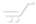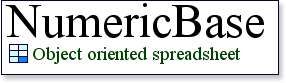Range formulas
Range formulas let you generate a range of values using just one formula. You can generate a range by defining a range formula at a cell located at the base row. NumericBase will generate values at the same column, and across the different rows of the table. The value of the cell that contain the range formula is a range message value.

There are two types of range formulas: numeric range, and data range.

Numeric range
You can specify a numeric range using several parameters:
• the starting number.
• the ending number.
• the number of steps.
• the step size.
The following screenshot shows a simple example - the numeric range is specified using the starting number (equals 5) ,and the ending number (equals 10).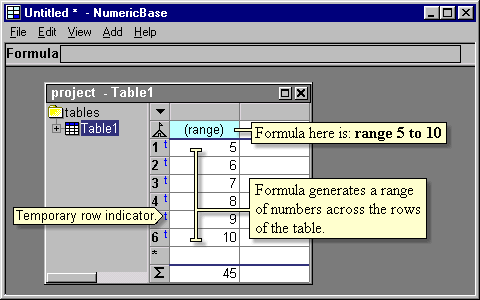Screenshot: range formula.

Numeric range syntax
The numeric range formula has 4 elements that appear in the following order:
1. The range keyword.
2. Formula. - specifies the first member of the range.
3. The to keyword.
4. Formula. - specifies the last member in the range.
For example: =range 5 to 10

optional clauses
The numeric range formula can have two optional clauses:

Step clause: Let you specify the step size of the numeric range. It has 2 elements that appear in the following order:
1. The step keyword
2. formula - specifies the step size of the range.
Steps clause: Let you specify the number of steps of the numeric range. It has 2 elements that appear in the following order:
1. The steps keyword
2. formula - specifies the number of steps in the range.
Using the "null constant" in a range formula.
You can use the null constant (represented by the question mark character) as one of the formulas in  range  formulas, NumericBase will deduce the values from the other parts of the formula.

Examples.

 Range formula Range values range 1 to 10 step 2 1,3,5,7,9 range 1 to 10 steps 2 1,10 range ? to 10 steps 2 step 1 9,10 range 3 to ? steps 2 step 2.5 3,5.5 range 1 to 10 step 1 steps 2 Will not generate a range because there are too many non-null parameters. Shows an appropriate error message.
Table: Numeric range examples.

Strong vs. weak range formula.
A "strong" range formula is a range formula that generates a fixed number of values. For example, the formula range 2 to 4 generate exactly 3 values : 2,3,and 4.

More example for strong range formulas are in the above table (all the range formulas there are strong range formulas)

A "weak" range formula do not have a fixed number of values. Instead, It depends on the number of rows of the table and generate values accordingly. For example, the formula range 1 to ? is a weak range formula. The following table shows different examples of weak range formulas.

 Formula Number of rows in the table Generated values range 1 to ? 2 1,2 range 1 to ? 4 1,3,4,5 range ? to 10 step 3 2 7,10 range ? to 10 step 3 3 4,7,10
Table: examples of weak ranges.

You can also see how a weak range depends on the number of rows in the table in the following screenshot (range formula is =range ? to 10 step 3 ). In the animated screenshot, we increase the number of rows by adding blank rows.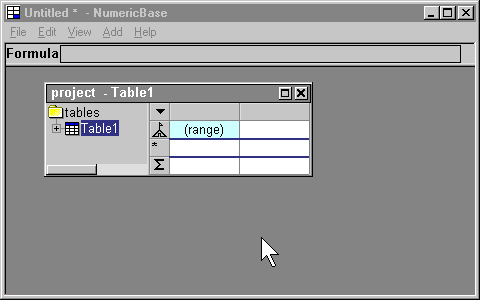Animated screenshot: Weak range depends on the number of rows in the table.

Using more than one strong range in a table
You can use more than one strong range formula in a table. In this case, NumericBase will show all the different combination of range values. See the following screenshot for an example.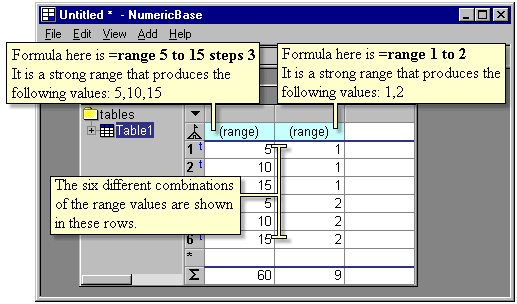Screenshot: Using two strong data ranges at the same table.

Data range
Data range let you generate a range of values from the existing values in a column. In this case, only values from cells that has formulas are considered. Also, values from the base row and summary row are not included, even if they have formulas. The syntax of this range has two elements that appear in the following order:
1. The "datarange" keyword
2. Formula that refers to a column, either in the same table or a different table.
For example:
1. =datarange a
2. =datarange Table.some_column
Usage in decision tables.
Data range is a "strong range". This is  because it have specific number of values - the number of cells that have formulas in the referred column. Therefore, if two data ranges are included in a table, the table will show all the possible combinations. This is useful, for example, for decision tables.

We have included an example of such a decision table. It is showing a hypothetical stock trading policy, and is located in the examples section (click here to get there).